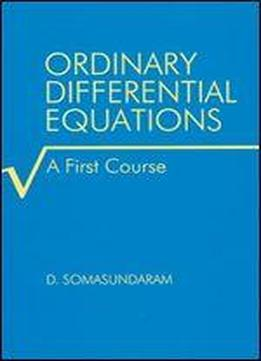# Ordinary Differential Equations: A First Course by D. Somasundaram / 2001 / English / PDF

Though ordinary differential equations is taught as a core course to students in mathematics and applied mathematics, detailed coverage of the topics with sufficient examples is unique. Written by a mathematics professor and intended as a textbook for third- and fourth-year undergraduates, the five chapters of this publication give a precise account of higher order differential equations, power series solutions, special functions, existence and uniqueness of solutions, and systems of linear equations. Relevant motivation for different concepts in each chapter and discussion of theory and problems-without the omission of steps-sets Ordinary Differential Equations: A First Course apart from other texts on ODEs. Full of distinguishing examples and containing exercises at the end of each chapter, this lucid course book will promote self-study among students.

views: 345